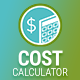How can I round up the total value and remove .00?

The best way to change the total value from e.g. 2500.00 to 2500 is to modify plugin’s code.

Please locate line 536 in cc.main.js in plugin’s folder. It should have the following code:

```total = total.toFixed( 2 ).replace( /(\\d)(?=(\\d{3})+\\.)/g, \'\$1,\' );
```

Replace the noted line with the following statement:

```total = Math.round(total);
```

It should round up your result to an integer without decimal points.